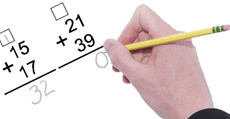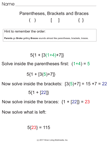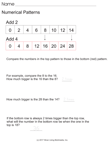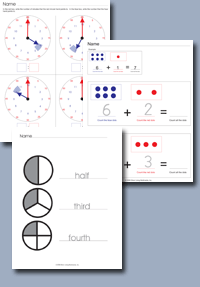﻿ Common Core Standards - Operations and Algebraic Thinking Grade 4Adaptive Worksheets

Helping Every Child to Succeed

toll-free (888) 777-0876
fax (888) 777-0875

## Operations and Algebraic Thinking for Grade 5

### Write and Interpret Numerical Expressions.

• CCSS.MATH.CONTENT.5.OA.A.1
Use parentheses, brackets, or braces in numerical expressions, and evaluate expressins with these symbols.
•Parentheses, Brackets and Braces

• CCSS.MATH.CONTENT.5.OA.A.2
Write simple expressions that record calculations with numbers, and interpret numerical expressions without evaluating them. For example, express the calculation "add 8 and 7, then multiply by 2" as 2 × (8 + 7). Recognize that 3 × (18932 + 921) is three times as large as 18932 + 921, without having to calculate the indicated sum or product.

• CCSS.MATH.CONTENT.5.OA.B.3
Generate two numerical patterns using two given rules. Identify apparent relationships between corresponding terms. Form ordered pairs consisting of corresponding terms from the two patterns, and graph the ordered pairs on a coordinate plane. For example, given the rule "Add 3" and the starting number 0, and given the rule "Add 6" and the starting number 0, generate terms in the resulting sequences, and observe that the terms in one sequence are twice the corresponding terms in the other sequence. Explain informally why this is so.Compare Numerical Patterns